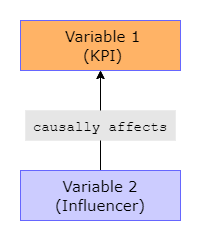# TIM Detect

This part of the documentation dives into TIM's capabilities for anomaly detection: TIM Detect. The following sections can be explored to build up a comprehensive understanding of these capabilities:

• How it works: how the solution is designed to go from raw data to accurate anomaly detection.
• KPI-driven: how TIM Detect's KPI-driven approach works and how to use it.
• Multivariate: how TIM Detect's multivariate approach works and how to use it.
• Concepts: how to prepare the experiment to achieve the best results for your problem.
• Glossary: the terminology of uncommon or specialized terms used in TIM Detect.

## The approaches​

TIM Detect solves time-series anomaly detection problems, supporting multidimensional data. Two approaches are available: the KPI driven approach and the multivariate approach.

Based on the data, problem, goal and preference, one or a combination is suitable. The KPI driven approach strives to detect whether a chosen variable (the KPI) behaves normally with respect to other variables (influencers), while the multivariate approach strives to detect whether the system (represented by all variables) is in a normal state. This means that what does and what does not constitute an anomaly can differ between the two approaches, as follows:

• for the KPI driven approach,
• an anomaly means the influencers are impacting the KPI less or more than expected, i.e. the actual state of the kPI would typically be higher or lower given the circumstances (influencers), while
• no anomaly means the influencers are impacting the KPI as usual, i.e. the actual value of the KPI is appropriate given the cirumstances (influencers); and
• for the multivariate approach,
• an anomaly means the current combination of all variables is unusual, i.e. the system is in an unknown state, whereas
• no anomaly means the current combination of all variables is expected, i.e. the system has often been found in a similar state before.

The following simple visual demonstration of a two-dimensional situation can in deciding which approach is right for a use case. It illustrates how the problem is understood by each approach (in the ideal scenario).To see the (differences in) results of both approaches, have a look at a simple two-dimensional dataset where variable 1 equals about half of variable 2.All data points are considered normal as Variable 1 is affected by Variable 2 as usual; all Variable 1 values are appropriate given the Variable 2 values.The point [10, 20] has an unusual combination of Variable 1 and Variable 2; the same or a similar combination of these variables was never seen before.

Which approach is more suited depends on the problem at hand, and should thus be decided case by case.